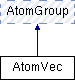ParM  parm A molecular dynamics library
AtomVec Class Reference

The main class for representing particles. More...

#include <box.hpp>

Inheritance diagram for AtomVec:## Public Member Functions

AtomVec (vector< double > masses)

AtomVec (uint N, flt mass)

AtomVec (AtomVec &other)

AtomVecvec ()

Atomoperator[] (cuint n)

Atomoperator[] (cuint n) const

AtomID get_id (cuint n)

uint size () const
Number of atoms in the group. More...

~AtomVec ()Public Member Functions inherited from AtomGroup
virtual Atomget (cuint n)

virtual AtomIter begin ()
For use in a for loop. More...

virtual AtomIter end ()

Vec com () const
center of mass More...

Vec com_force () const
center of mass force (i.e., sum of all forces) More...

Vec com_velocity () const
center of mass velocity More...

flt mass () const
Mass of the whole group. More...

flt kinetic_energy (const Vec originvelocity=Vec::Zero()) const
Total kinetic_energy energy of the group. More...

Vec momentum () const
Total momentum. More...

$$R_g$$ More...

Vec torque (const Vec loc) const
Total torque about a given location. More...

Vec torque () const

flt moment_about (const Vec axis, const Vec loc) const
Moment of inertia of the atoms about an axis. More...

flt moment_about (const Vec axis) const

Vec angular_momentum (const Vec loc) const
Angular momentum. More...

Vec angular_momentum () const

Matrix moment (const Vec loc) const
Moment of inertia of the atoms as a whole. More...

Matrix moment () const

Vec omega (const Vec loc) const
Angular velocity. More...

Vec omega () const

void add_omega (Vec w, Vec origin)
Add a given angular velocity to all atoms, by adding to their velocity. More...

void reset_L ()
Reset angular momentum to 0. More...

for resetting. Adds a fixed velocity to all atoms More...

void reset_com_velocity ()
Subtracts the center of mass velocity from all atoms. More...

void randomize_velocities (flt T)
Randomize velocities, for a specific temperature. More...

void reset_forces ()
for timestepping More...

virtual ~AtomGroup ()

## Detailed Description

The main class for representing particles.

Examples:
hardspheres.cpp, LJatoms.cpp, and packer.cpp.

## Constructor & Destructor Documentation

 AtomVec::AtomVec ( vector< double > masses )
inline
 AtomVec::AtomVec ( uint N, flt mass )
inline
 AtomVec::AtomVec ( AtomVec & other )
inline
 AtomVec::~AtomVec ( )
inline

## Member Function Documentation

 AtomID AtomVec::get_id ( cuint n )
inlinevirtual

Implements AtomGroup.

Examples:
hardspheres.cpp, LJatoms.cpp, and packer.cpp.
 Atom& AtomVec::operator[] ( cuint n )
inlinevirtual

Implements AtomGroup.

 Atom& AtomVec::operator[] ( cuint n ) const
inlinevirtual

Implements AtomGroup.

 uint AtomVec::size ( ) const
inlinevirtual

Number of atoms in the group.

Implements AtomGroup.

Examples:
hardspheres.cpp, LJatoms.cpp, and packer.cpp.
 AtomVec& AtomVec::vec ( )
inlinevirtual

Implements AtomGroup.

The documentation for this class was generated from the following file: